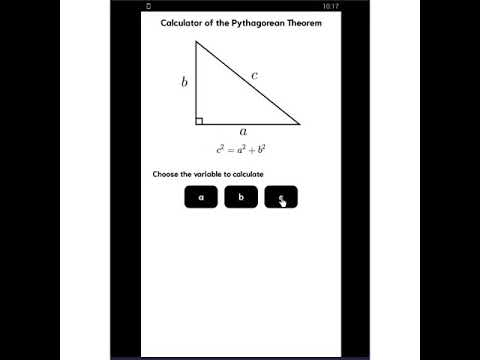# Trigonometry assistant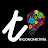50K+Everyone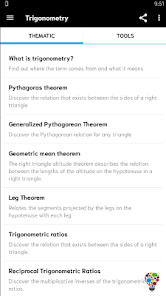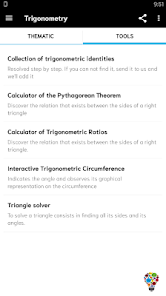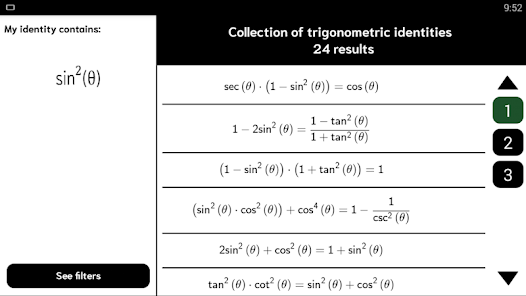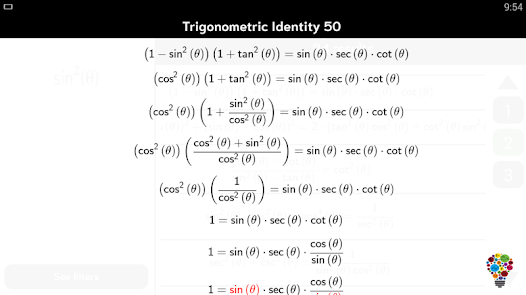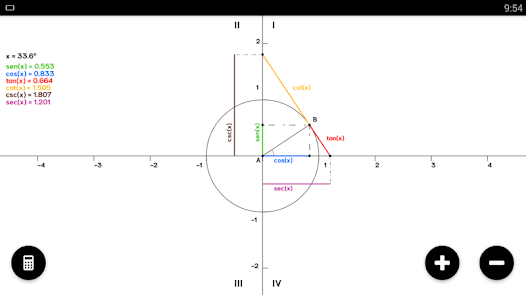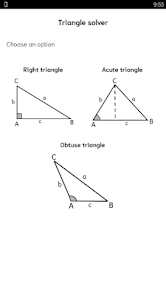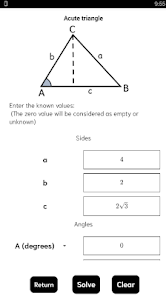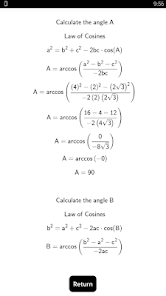The purpose of this application is to become a powerful tool in the solution of mathematical exercises on trigonometry

It contains the following Tools:

* Collection of Trigonometric Identities, resolved step by step. Does not the one you were looking for appear? Please send it and we will add it in future updates.

* Triangles Solver. Tell us the sides and angles that you know of a triangle and you will get the solution step by step of it.

* Calculator of the Pythagorean Theorem. Indicate the side of the right triangle you want to calculate and you will see its detailed solution.

* Calculator of Trigonometric Ratios. Give us the angle or sides of the triangle and we will calculate the 6 trigonometric ratios.

* Interactive Trigonometric Circumference. Indicate the angle or click on the touch of your smartphone to visualize the trigonometric lines instantly.

In addition to this the App contains the following theoretical contents of support for you:

* What is Trigonometry?
* Pythagoras theorem
* Generalized Pythagorean Theorem
* Geometric mean theorem
* Leg Theorem
* Trigonometric Ratios
* Trigonometric Ratios of StandardAngles
* Trigonometric Identities
Updated on
Feb 26, 2023

## Data safety

Safety starts with understanding how developers collect and share your data. Data privacy and security practices may vary based on your use, region, and age. The developer provided this information and may update it over time.No data shared with third parties